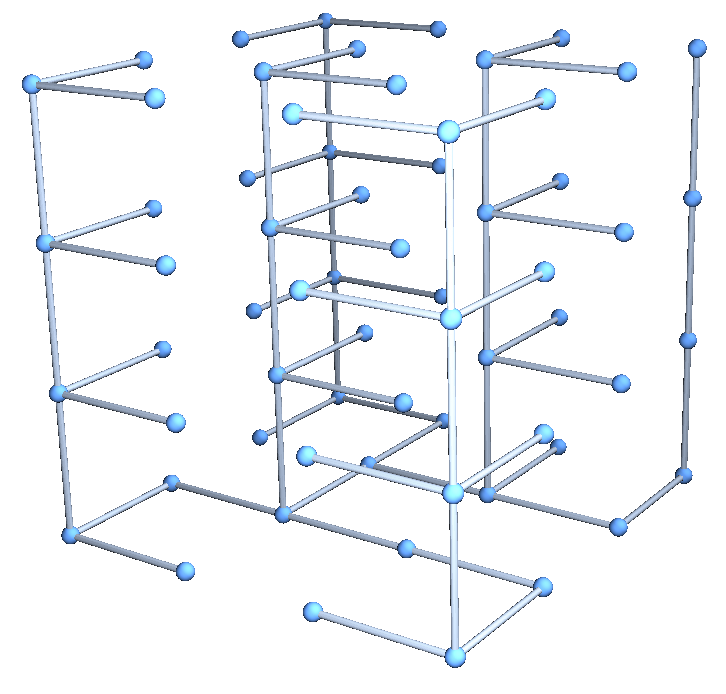# KruskalAlgorithm

Contributed by: Ed Pegg Jr

Find the minimal spanning tree for a given set of edges

 ResourceFunction["KruskalAlgorithm"][edges] finds the minimal spanning tree for a graph given by edges. ResourceFunction["KruskalAlgorithm"][edges,"Graph"] finds the minimal spanning tree and shows the graph.

## Examples

### Basic Examples

Return a minimal spanning tree for a given set of points:

 In:=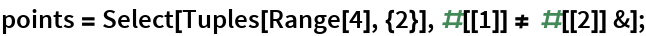In:=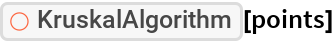Out=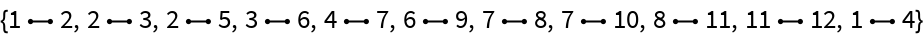### Scope

If “Graph” is specified, show the Graph:

 In:=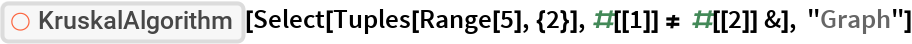Out=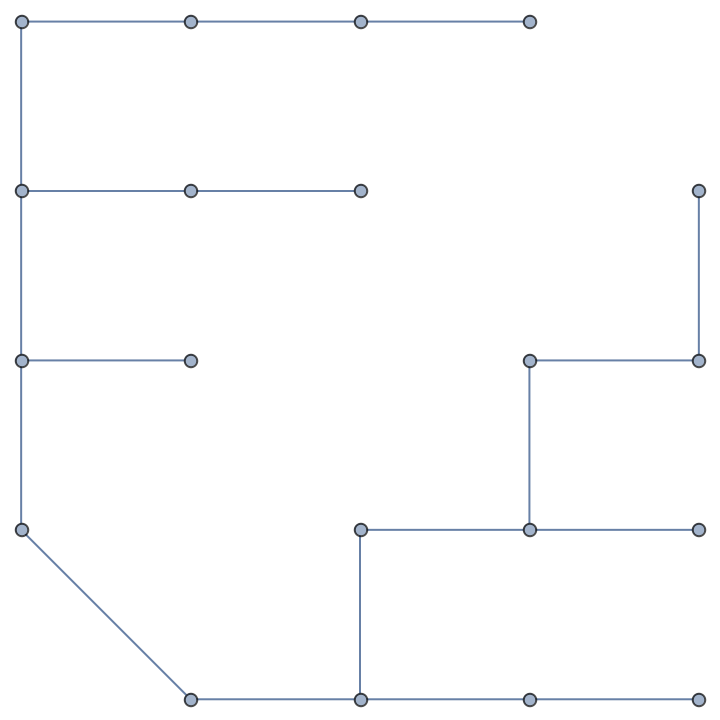### Possible Issues

The minimal spanning tree is not necessarily unique:

 In:=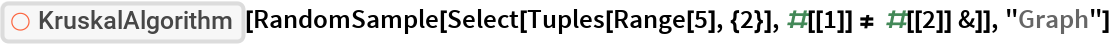Out=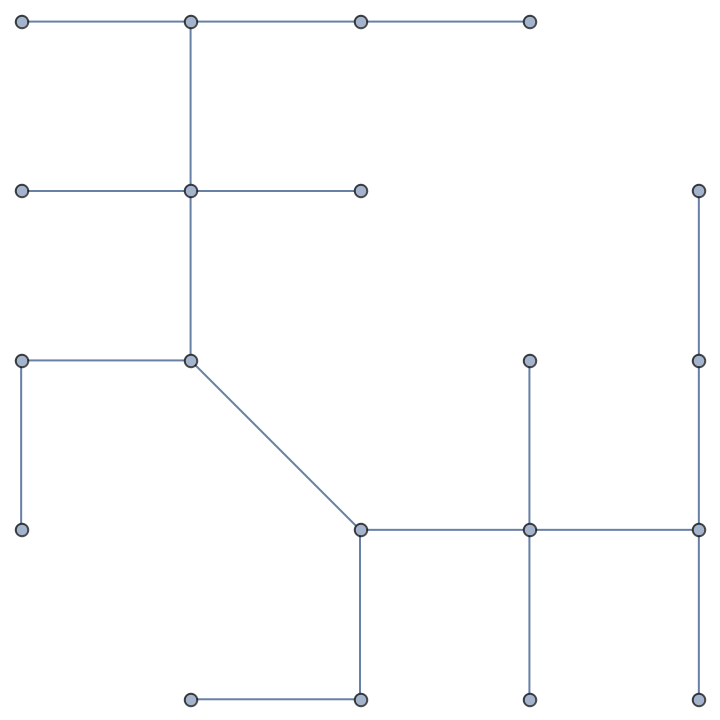### Neat Examples

 In:=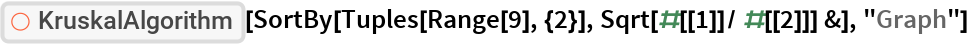Out=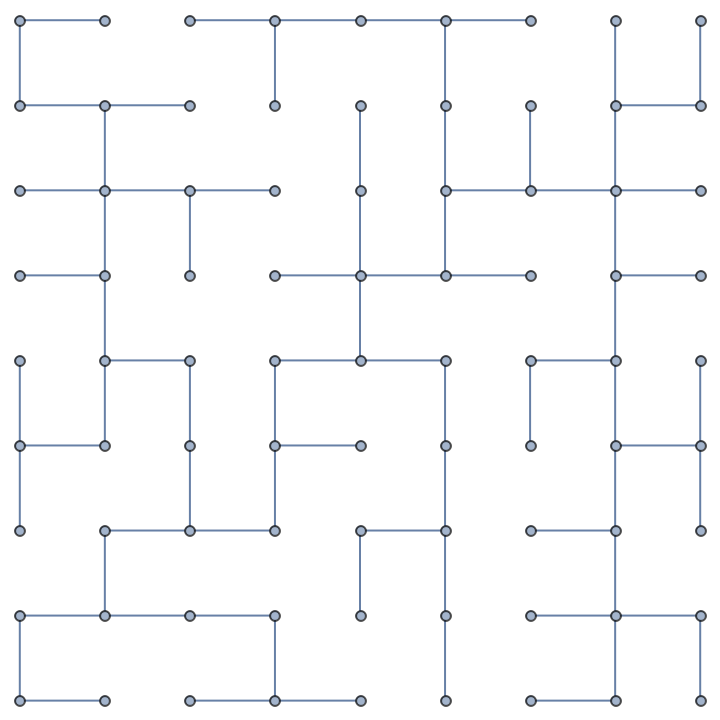In:=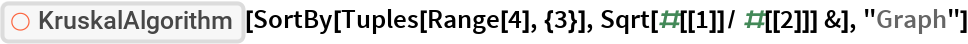Out=